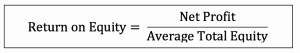# Profitability Ratios

## Introduction

Profitability ratios are the ratios that are used to measure the company’s ability to generate income or profit during a period of time. Profitability refers to the company’s ability to generate income or profit during a period of time. Usually, profitability is one of the most important measures of the company’s success because it usually links directly to the main objective of the company itself, which is “Profit”.

The company usually uses the profitability ratios in measuring its performance during the period. However, its profitability ratios alone in a single period may not be able to tell much how the company is doing. Hence, they are usually used by comparing with the prior period or with other companies in the same industry. This way they will show a much better picture of how the company is doing.

## 5 Types of Profitability RatioThe five main types of profitability ratios include:

1. Gross Profit Margin
2. Operating Profit Margin
3. Net Profit Margin
4. Return on Assets
5. Return on Equity

All the above ratios, the higher they are, the better the company’s performance is when compared with the prior period or with other companies in the same industry.

### Gross Profit Margin

Gross profit margin, also known as gross margin is the ratio that is used to measure the company’s profitability at the direct trading level. It shows the percentage remaining from the revenues, after deducting the cost of goods sold.

Gross profit margin can be increased by increasing the selling price, increasing the sales volume or reducing the cost (by better negotiating with suppliers or finding lower-cost suppliers, etc.)

#### Formula of Gross Profit Margin

Gross profit margin can be calculated by taking the remaining of revenues, after deducting the cost of goods sold, comparing to revenues.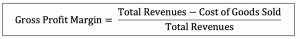### Operating Profit Margin

Operating profit margin, also known as operating margin is the ratio that is used to measure the company’s profitability at the operating level. It shows the percentage remaining from the revenues, after deducting the cost of goods sold and operating expenses.

Operating profit margin shows how well the company performs in managing its operating expenses. However, the low operating profit margin is not necessary due to the poor managing of the operating expenses by the company. It can be due to the other factors such as selling price, sale volume or the cost of materials, etc.

Operating profit margin can be increased by reducing operating expenses, increasing the selling price, increasing the sales volume, or reducing the cost.

#### Formula of Operating Profit Margin

Operating profit margin can be calculated by comparing operating income or operating profit, profit before interest and tax of the company, to total revenues.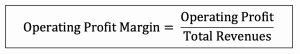*Operating profit = Profit before interest and tax

### Net Profit Margin

Net profit margin, also known as profit margin or net margin, is the ratio that is used to measure the company’s profitability by comparing the net profit or net income to the total revenues. It shows the company’s ability in managing costs and expenses in a period of time.

Profit margin shows the percentage remaining from the total revenues, after deducting all the costs and expenses including interest and tax.

#### Formula of Net Profit Margin

Net profit margin can be calculated by comparing net income or net profit after interest and tax to total revenues.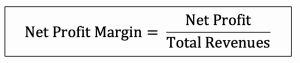### Return on Assets

Return on assets is the ratio that is used to measure the company’s ability to generate profit by using its whole resource, the assets. It shows the percentage of the net income or net profit comparing to the average total assets.

Return on assets shows how efficient the company is in using the assets to generate profits in a period of time. The high return on assets usually shows that the company performs well in making a profit from the assets it has.

#### Formula of Return on Assets

Return on assets can be calculated by comparing net income or net profit after interest and tax in the period to average total assets.

##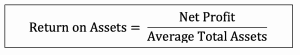### Return on Equity

Return on equity is the ratio that is used to measure the company’s ability to generate profit by using its investors’ money. It shows the percentage of the net income or net profit comparing to the average total equity.

Return on equity shows how efficient the company is in using the investor’s money to generate profits in a period of time. The high return on equity usually shows that the company performs well in making profits from its investors’ money.

#### Formula of Return on Equity

Return on equity can be calculated by comparing net income or net profit after interest and tax in the period to average total equity.